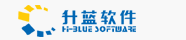Customer Relationship Management

# 如何实现给定日期的若干天以后的日期(有点类似VB中的D

March 25,2004

http://www.asp888.net 豆腐技术站

function CheckRun(\$year){
if(\$year%4==0 && (\$year%100!=0 || \$year%400==0) )
return true;
else
return false;
}

\$parts = explode(' ', \$date);
\$date = \$parts;
\$time = \$parts;
\$ymd = explode('-', \$date);
\$hms = explode(':', \$time);
\$year = \$ymd;
\$month = \$ymd;
\$day = \$ymd;
\$hour = \$hms;
\$minute = \$hms;
\$second = \$hms;
\$day=\$day+1 ; //废话少说，先把日期加一再说
if(\$month=='1' || \$month=='3' || \$month=='5' || \$month=='7' || \$month=='8' || \$month=='10' || \$month=='12')
if(\$day==32)
{
\$day='1';
\$month++;
}
if(\$month=='4' || \$month=='6' || \$month=='9' || \$month=='11')
if(\$day==31)
{
\$day='1';
\$month++;
}
if(\$month=='2')
if(CheckRun(\$year))
{
//闰年 2月有 29 天
if(\$day==30)
{
\$day=1;
\$month++;
}
}
else
{
//不是闰年
if(\$day==29)
{
\$day=1;
\$month++;
}
}
if(\$month==13)
{
\$month=1;
\$year++;
}
return \$year . "-" . \$month . "-" . \$day;
}